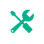# Long Division Calculator

Long Division Calculator
1. 1. Long Division Calculator
2. 2. Long Division Calculator
3. 3. Long Division Calculator
LCM of
302
300 = 2 x 2 x 3 x 5 x 5
300 = 2 x 2
300 = 2 x 2 x 3
300 = 22 x 3 x 52
30 = 2 x 3 x 5
= 22 x 52 x 3
= 4 x 25 x 3
LCM(300, 30) = 300
 2 30 300 2 15 150 3 15 75 5 5 25 5 1 5 1 1
2 * 2 * 3 * 5 * 5
LCM = 300
300: 300 600 900
300: 300 600 900 600 900 600 900 600 900 600 900 600 900
LCM(300,30) = 300

## LCM Calculator

The online LCM calculator can find the least common multiple (factors) quickly than manual methods. Whether finding the LCM of two numbers or multiple numbers, this calculator can help you with just a single click.Related Tool Name

Long Division calculator